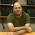## Monday, July 25, 2011

### Autocorrelation in Bayesian ANOVA: Fixing the book's overkill

In hierarchical Bayesian ANOVA (Ch's 18, 19, and 22 of the book), the model expresses the cell means in terms of deflections from baseline, such that the deflections sum to zero. The sum-to-zero constraint is only imposed after BUGS does its work; i.e., the deflection parameters in the BUGS model are not intrinsically constrained to sum to zero. The deflection parameters in the model can therefore become strongly autocorrelated, and extensive thinning may be necessary to attenuate autocorrelation in the unconstrained parameters.

But we don't care about the unconstrained parameters. What we care about is the sum-to-zero versions of the parameters, because those are the parameters we use to interpret the data. It turns out that autocorrelation in the sum-to-zero parameters is much less severe. I've known about this for quite a while, but haven't gotten around to modifying the programs until now.

Here's an example. This comes from the two-factor hierarchical Bayesian ANOVA in Section 19.1.3 (p. 520) of the book, regarding salaries. The deflection parameters in the BUGS model are labeled a1[], a2[], and a1a2[,]. The sum-to-zero versions of the parameters are labeled b1[], b2[], and b1b1[,]. Here are the a1[] chains when the thinning is set to 75:

Obviously there is nasty autocorrelation, and much more thinning would be needed if we cared about these parameters. (Added Nov. 7, 2011: Regarding thinning, see a later post which points out that thinning is not advisable. But the remainder of this post, below, is still important for better interpretation of the parameters in Bayesian ANOVA.)

But we don't care about a1[], instead we care about the sum-to-zero reparameterization, b1[]. Here are the b1[] chains derived from the chains above:

There is minimal autocorrelation in the sum-to-zero versions of the parameters.

Therefore, the versions of the programs that accompany the book, which assess and attenuate autocorrelation in the non-sum-to-zero parameters, thin far more than is necessary. The results are not wrong, merely overkill.

I have created new versions of two of the ANOVA programs such that the sum-to-zero constraint is computed inside the BUGS model statement, and therefore the sum-to-zero parameters can be assayed using the plotChains() command. The two new programs are ANOVAonewayBRugsSTZ.R and ANOVAtwowayBRugsSTZ.R . These programs use far less thinning than the original programs to achieve the same results. Other ANOVA programs --such as those that allow heterogeneous variances, and Poisson exponential ANOVA-- can be modified analogously.

1.2.